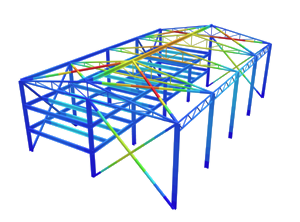SkyCiv Documentation

Your guide to SkyCiv software - tutorials, how-to guides and technical articles

1. Home
2. Tutorials
3. Beam Tutorials
4. How to Calculate Reactions at Supports?

# How to Calculate Reactions at Supports?

## How to Calculate the Reactions at the Supports of a Beam

This is always the first step in analyzing a beam structure, and it is generally the easiest. It involves calculating the reaction forces at the supports (supports A and B in the below example) due to the forces acting on the beam. You will need to know this to progress through and calculate bending moment diagrams (BMDs) and shear force diagrams (SFDs); an important part of your statics and structural college/university courses. SkyCiv offers a powerful reaction calculator that allows you to model any beam and show these hand calculations for you, but it is also an important concept to understand.

When solving a problem like this we want to first remember that the beam is static; meaning it is not moving. From simple physics, this means that the sum of the forces in the y direction equals zero (i.e. the total downward forces equal the total upward forces). A second formula to remember is that the sum of the moments about any given point is equal to zero. This is because the beam is static and therefore not rotating.

To determine the reactions at supports, follow these simple steps:

### 1. Let the sum of moments about a reaction point equal to ZERO (ΣM = 0)

All we need to know about moments at this stage is that they are they are equal to the force multiplied by the distance from a point (i.e. the force x distance from a point).

Consider a simple example of a 4m beam with a pin support at A and roller support at B. The free body diagram is shown below where Ay and By are the vertical reactions at the supports: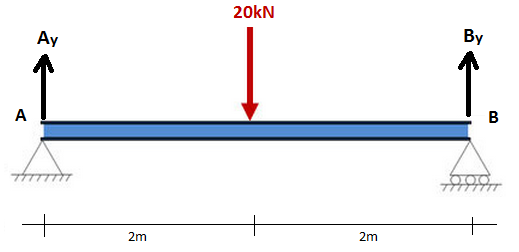We firstly want to consider the sum of moments about point B and let it equal zero. We have chosen point B to prove this can be done at either end of the beam (provided it is pin supported). However, you could just as easily work from point A. So, now we sum the moments about point B and let the sum equal 0: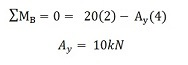NOTE: The sign convention we have chosen is that counter-clockwise moment are positive and clockwise moments are negative. This is the most common sign convention but it is up to you. You must ALWAYS use the same sign convention throughout the whole problem. Always use the same sign convention from the start. We now have our first equation. We need to solve another equation in order to find By (the vertical reaction force at support B).

### 2. Let the sum of vertical forces equal to 0 (ΣFy = 0)

Sum the forces in the y (vertical) direction and let the sum equal zero. Remember to include all forces including reactions and normal loads such as point loads. So if we sum the forces in the y direction for the above example, we get the following equation: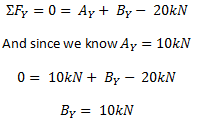NOTE: Again we stuck to a sign convention which was to take upward forces (our reactions) as positive and downward forces (the point load) as negative. Remember the sign convention is up to you but you must ALWAYS use the same sign convention throughout the whole problem.

So there we have it, we have used the two above equations (sum of moments equals zero and sum of vertical forces equals zero) and calculated that the reaction at support A is 10 kN and the reaction at support B 10kN. This makes sense as the point load is right in the middle of the beam, meaning both supports should have the same vertical forces (i.e. it is symmetric).

In summary, here are the full hand calculations produced by SkyCiv Beam: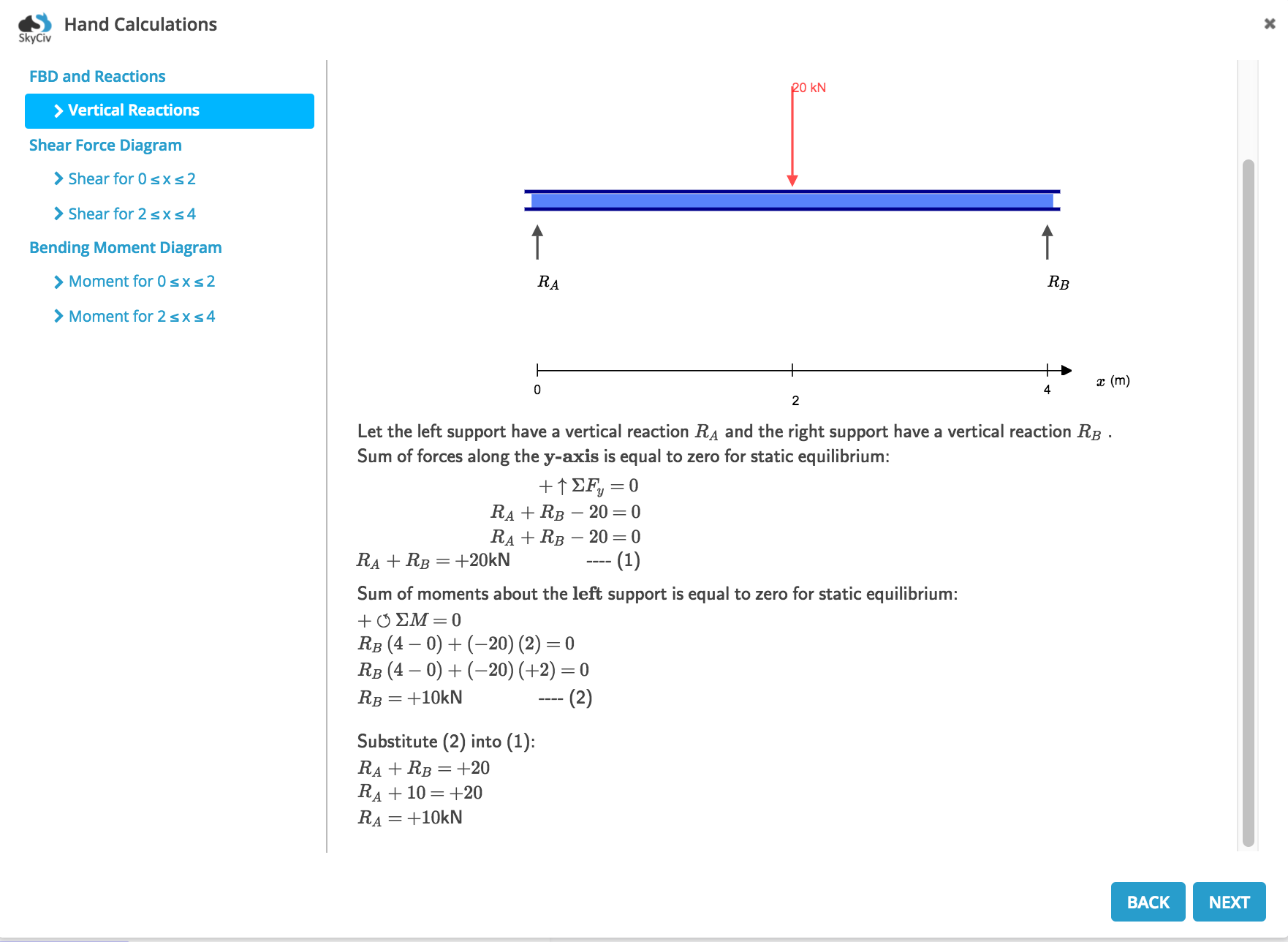Hand Calculations from SkyCiv Beam